NCERT Solutions Chapter 3 - Coordinate Geometry, Class 9, Maths

# NCERT Solutions Chapter 3 - Coordinate Geometry, Class 9, Maths - Extra Documents & Tests for Class 9

1. How will you describe the position of a table lamp on your study table to another person?

To describe the position of a table lamp on the study table, we have two take two lines, a perpendicular and horizontal. Considering the table as a plane and taking perpendicular line as Y axis and horizontal as X axis. Take one corner of table as origin where both X and Y axes intersect each other. Now, the length of table is Y axis and breadth is X axis. From The origin, join the line to the lamp and mark a point. Calculate the distance of this point from both X and Y axes and then write it in terms of coordinates.
Let the distance of point from X axis is x and from Y axis is y then the the position of the table lamp in terms of coordinates is (x,y).

2. (Street Plan) : A city has two main roads which cross each other at the centre of the city. These two roads are along the North-South direction and East-West direction.
All the other streets of the city run parallel to these roads and are 200 m apart. There are 5 streets in each direction. Using 1cm = 200 m, draw a model of the city on your notebook. Represent the roads/streets by single lines. There are many cross- streets in your model. A particular cross-street is made by two streets, one running in the North - South direction and another in the East - West direction. Each cross street is referred to in the following manner : If the 2nd street running in the North - South direction and 5 th in the East - West direction meet at some crossing, then we will call this cross-street (2, 5). Using this convention, find:
(i) how many cross - streets can be referred to as (4, 3).
(ii) how many cross - streets can be referred to as (3, 4)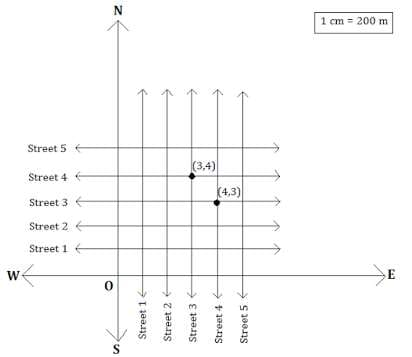(i) Only one street can be referred to as (4, 3) as we see from the figure.

(ii) Only one street can be referred to as (3, 4) as we see from the figure.

Exercise 3.2

1. Write the answer of each of the following questions:

(i) What is the name of horizontal and the vertical lines drawn to determine the position of any point in the Cartesian plane?

(ii) What is the name of each part of the plane formed by these two lines?

(iii) Write the name of the point where these two lines intersect.

(i) The name of horizontal lines and vertical lines drawn to determine the position of any point in the Cartesian plane is x-axis and y-axis respectively.

(ii) The name of each part of the plane formed by these two lines x-axis and y-axis is quadrants.

(iii) The point where these two lines intersect is called origin.

2. See Fig.3.14, and write the following:

(i) The coordinates of B.
(ii) The coordinates of C.
(iii) The point identified by the coordinates (-3, -5).
(iv) The point identified by the coordinates (2, -4).
(v) The abscissa of the point D.
(vi) The ordinate of the point H.
(vii)The coordinates of the point L.
(viii) The coordinates of the point M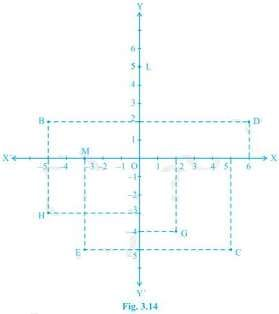(i) The coordinates of B is (-5, 2).

(ii) The coordinates of C is (5, -5).

(iii) The point identified by the coordinates (-3, -5) is E.

(iv) The point identified by the coordinates (2, -4) is G.

(v) Abscissa means x coordinate of point D. So, abscissa of the point D is 6.

(vi) Ordinate means y coordinate of point H. So, ordinate of point H is -3.

(vii) The coordinates of the point L is (0, 5).

(viii) The coordinates of the point M is (- 3, 0).

Exercise 3.3

1. In which quadrant or on which axis do each of the points (-2, 4), (3, -1), (-1, 0), (1, 2) and (-3, -5) lie? Verify your answer by locating them on the Cartesian plane.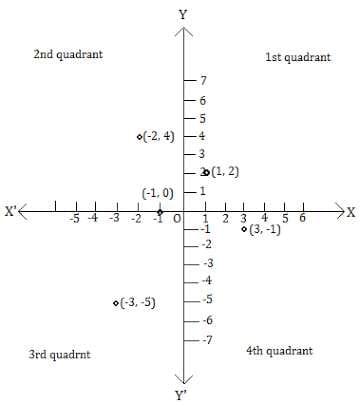2. Plot the points (x, y) given in the following table on the plane, choosing suitable units of distance on the axes.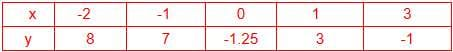Points (x,y) on the plane. 1unit = 1 cm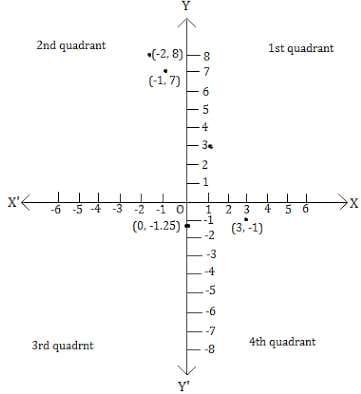The document NCERT Solutions Chapter 3 - Coordinate Geometry, Class 9, Maths | Extra Documents & Tests for Class 9 is a part of the Class 9 Course Extra Documents & Tests for Class 9.
All you need of Class 9 at this link: Class 9

## Extra Documents & Tests for Class 9

1 videos|228 docs|21 tests

## FAQs on NCERT Solutions Chapter 3 - Coordinate Geometry, Class 9, Maths - Extra Documents & Tests for Class 9

 1. What is the importance of coordinate geometry in mathematics?Ans. Coordinate geometry is important in mathematics as it provides a way to study and analyze geometric figures using algebraic techniques. It helps in solving problems related to distances, angles, and equations of lines and curves. It also has applications in various fields such as physics, engineering, computer science, and architecture.
 2. How do we find the distance between two points in coordinate geometry?Ans. To find the distance between two points in coordinate geometry, we can use the distance formula. The distance formula is derived from the Pythagorean theorem and is given by: Distance = √[(x2 - x1)^2 + (y2 - y1)^2] Here, (x1, y1) and (x2, y2) are the coordinates of the two points. By substituting the values of the coordinates into the formula, we can calculate the distance between the two points.
 3. What is the slope of a line in coordinate geometry?Ans. The slope of a line in coordinate geometry represents the steepness of the line. It is a measure of how much the line rises or falls for a given horizontal distance. The slope of a line passing through two points (x1, y1) and (x2, y2) is given by the formula: Slope = (y2 - y1) / (x2 - x1) The slope can be positive, negative, or zero, indicating whether the line is rising, falling, or horizontal, respectively. It helps in determining the direction and inclination of the line.
 4. How do we find the equation of a line in coordinate geometry?Ans. To find the equation of a line in coordinate geometry, we need to know either the slope of the line and a point on the line or two points on the line. If we know the slope, denoted by m, and a point (x1, y1) on the line, we can use the point-slope form of the equation: y - y1 = m(x - x1) If we know two points (x1, y1) and (x2, y2) on the line, we can use the slope-intercept form of the equation: y = mx + c where m is the slope and c is the y-intercept, which can be calculated using the formula: c = y1 - mx1 Substituting the values into the respective equations, we can determine the equation of the line.
 5. How do we determine the area of a triangle using coordinate geometry?Ans. To determine the area of a triangle using coordinate geometry, we can use the formula for the area of a triangle formed by three points (x1, y1), (x2, y2), and (x3, y3). The formula is given by: Area = 1/2 * |x1(y2 - y3) + x2(y3 - y1) + x3(y1 - y2)| Here, the absolute value is taken to ensure a positive area. By substituting the coordinates of the three points into the formula, we can calculate the area of the triangle.

## Extra Documents & Tests for Class 9

1 videos|228 docs|21 testsExplore Courses for Class 9 examSignup to see your scores go up within 7 days! Learn & Practice with 1000+ FREE Notes, Videos & Tests.
10M+ students study on EduRev
Track your progress, build streaks, highlight & save important lessons and more!
Related Searches

,

,

,

,

,

,

,

,

,

,

,

,

,

,

,

,

,

,

,

,

,

,

,

,

,

,

,

;